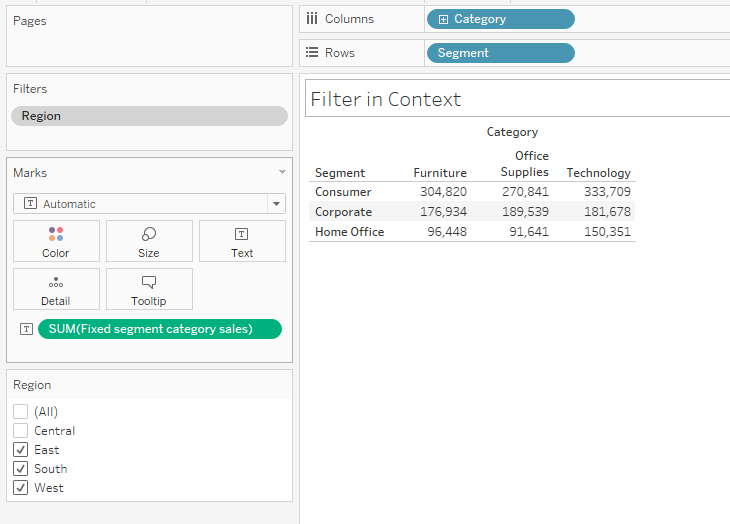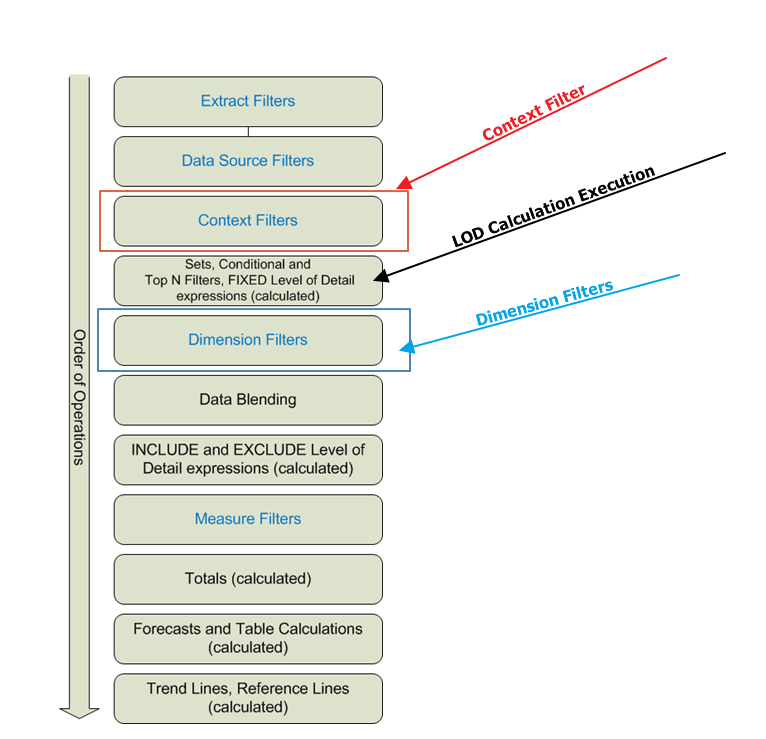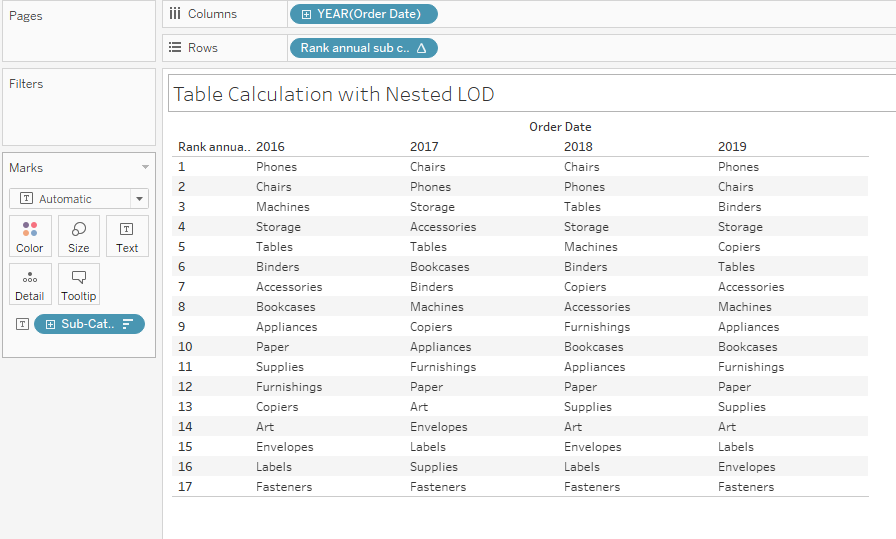Jim Dehner

Tableau How to’s,  Use Cases, and Forums Questions

Hey #DataFam

Let’s Talk

Open for

## Need help using LOD’s –

Think of your data set as a pyramid.  The data you upload creates the base of the pyramid – the top is the sum of all the data.  LOD expressions give you a way to create layers in your data and use those layers to categorize data into dynamic groups, sets, or ways to work around the limitations of table calculations –

## LOD Syntax

LODs use a keyword – Fixed, Include, or Exclude – followed by a list of dimensions that set the level of the virtual layer you want to create in the data set –  For example

{Fixed  [Segment], [Category]    : …

In words it says “make all the combinations of Segment (3 in Superstore data)  and Category (3) ”  and create a layer that consists of 9 total values – then save them so they can be used later

The colon :   is the divider that ends the layer definition and starts the formula of the value to save –

{Fixed  [Segment], [Category]    :   sum(Sales)}

The result is a new layer in the data at the Segment/Category level## Creating multiple layers

Adding dimensions before the colon will define another layer in the data.  To create a new layer that includes Year level place the Year(order date) in the dimension list

{ FIXED [Segment],[Category],year([Order Date]): sum([Sales])}

Now in addition to layer at the Segment/Category level, there is a layer at the Segment/Category/Year level## Filtering and the Order of Operation

LOD expressions can be filtered but the results will be affected if dimensions placed in the Context Filter

First, use a simple filter on a Dimension in the LOD expression – ( Segment ). The results are filtered as you would expect – the Consumer Segment is filtered out of the data – Three of the 9 values of the Segment/Category are filtered out of the result## Context filters affect

Add a filter for a Dimension not in the LOD expression Region in the example – filter the Central region and the 9 values from the LOD are NOT changed –Change the Region filter to Context – the results do changeWhat's going on?Context filters are applied BEFORE the LOD calculation in the Order of Operation – if a dimension is placed in Context Tableau will apply the filter before the LOD is calculated –

In this case – the Central Region is filtered out before calculating the Segment/Category LOD

Dimension filters are applied AFTER the LOD is calculated – the results reflect the un-filtered Segment/Category LOD

## Use in other calculations

LODs place a value that is aggregated in a new virtual layer in the data set, but they are NOT aggregates in themselves.   That’s powerful!

## Replacing Table calculations

A FAQ on the Forum is how to fix a table calculation – but they are built on the table that underlays each worksheet and can’t be carried from one sheet to another.

LOD’s can be used to "Fix" some table calculations – for example, Percent of total

The table calculation formula for percent of total used Total() and is then calculated down (in this example)

SUM([Sales]) / TOTAL(SUM([Sales]))

LOD’s can be used to replace the numerator and denominator

sum({ FIXED [Segment],[Category]: sum([Sales])})/sum({ FIXED [Category]: sum([Sales])})The LOD base Percent of Total and now Fixed at the Segment/Category level

## Nested LOD and Table Calculation

LODs can be also be nested directly in Table Calculations – This example dynamically ranks Sub-Category based on annual sales

The LOD below will total Sales at the Sub-Category/Year level

{ FIXED [Sub-Category],Year([Order Date]):sum([Sales])}

Nesting the LOD in a Ranking function (Table Calculation)  will sequence the categories descending each year

RANK_UNIQUE(sum([fixed annual category sales]),'desc')

And return this#### LODs in conditional statements and grouping

Need to separate records into groups based on the value of a single dimension?

Use a conditional statement (  if…then  ) in the aggregation portion of the LOD  (after the :  )
This says “for each order and category combination – separate out the Furniture lines and group the remaining as “Other”

{ FIXED [Order ID],[Category] : min(if [Category]="Furniture" then [Category] else "Other" end  ) }

The LOD will categorize each Order/Category combination into one of 2 groups – you can use the LOD like any other dimension in your viz#### Include and Exclude LODs

Where Fixed works above the dimension filters in the order of operations Include and Exclude work after the dimension have been applied

Using the same Segment/Category but adding the Include and Exclude version we can see the effect of the LOD

{ FIXED [Segment],[Category]: sum([Sales])}

{ EXCLUDE   [Segment],[Category]: sum([Sales])}

{ INCLUDE  [Segment],[Category]: sum([Sales])}

Include is applied after the dimension filter  Year(order date) is applied and sums at the  Segment/Category for each year

Exclude applies the totals after the Year(order date) is applied and sums across all the Segment/Categories at the year levelI hope this gives you a better idea of how LOD expressions give you the flexibility to add different levels in your data set.  They are a powerful tool that can be used in conjunction with conditional statements, table calculations or nested in other LODs.

Have fun – the best way to learn more is to use them with some simple example

A Tableau workbook with copies of the examples presented here can be found on my tableau public site   https://public.tableau.com/profile/jim.dehner#!/vizhome/LayeryourdatawithLODs/FixedSegCatyrsumsales

Jim